##### Search### KCSE CLUSTER TESTS 15

#### Physics Paper 3

1.

You are provided with the following apparatus

- Two metre rules (one metre rule and half metre rule)

- Two stands and clamps.

- Two bosses.

- Three pieces of threads ( at least 1m, 30cm long)

- A spring

- A piece of cellotape or a plasticine

- One mass 200g

- A stop watch

- Optical pin
Proceed as follows

i) Set up the apparatus as shown in the figure below. Attach the pin (pointer) at one end of the metre rule using cellotape.ii) Suspend one end of the metre rule with a thread at 5cm mark from the other end.

iii) Suspend the other end with a spring also 5cm from the end so that the metre rule is horizontal.

iv) Hold the other rule vertically on the bench so that it is near the end with a pointer as shown in the diagram above. v) Read the pointer position, Lo =................................................cm (1mk)

vi) Hang on the horizontal metre rule, the 200g mass at a length, L= 10cm from the spring. Record the extension, e of the spring in table below.

vii) Displace the mass slightly downwards and release it to oscillate vertically. Take time for 20 oscillations and record in the table below.

viii) Repeat for the position of L, of the mass.

ix) Plot a graph of extension e (cm) against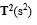x) Calculate the slope, R of the graph. (3mks)

xi) Given that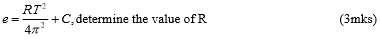Complete the table below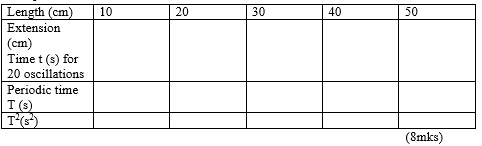20 marks

2.

You are provided with the following apparatus

- A wire W mounted on a mm scale

- One dry cell.

- A switch

- An ammeter

Proceed as follows

a) Connect the circuit as shown in the figure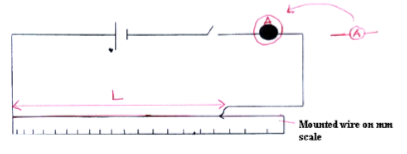b) i) Adjust the distance L of the wire to 20cm.

ii) Close the switch and record the ammeter reading.

iii) Repeat the procedure above for values of L shown on the table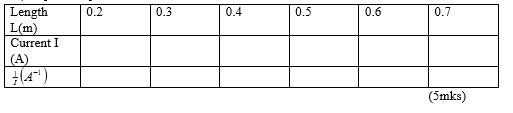a) Plot a graph of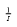against length L (m) (5mksb) Determine the value of the slope S of the graph (2mks)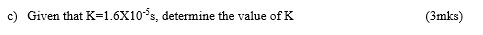20 marks

Back Top